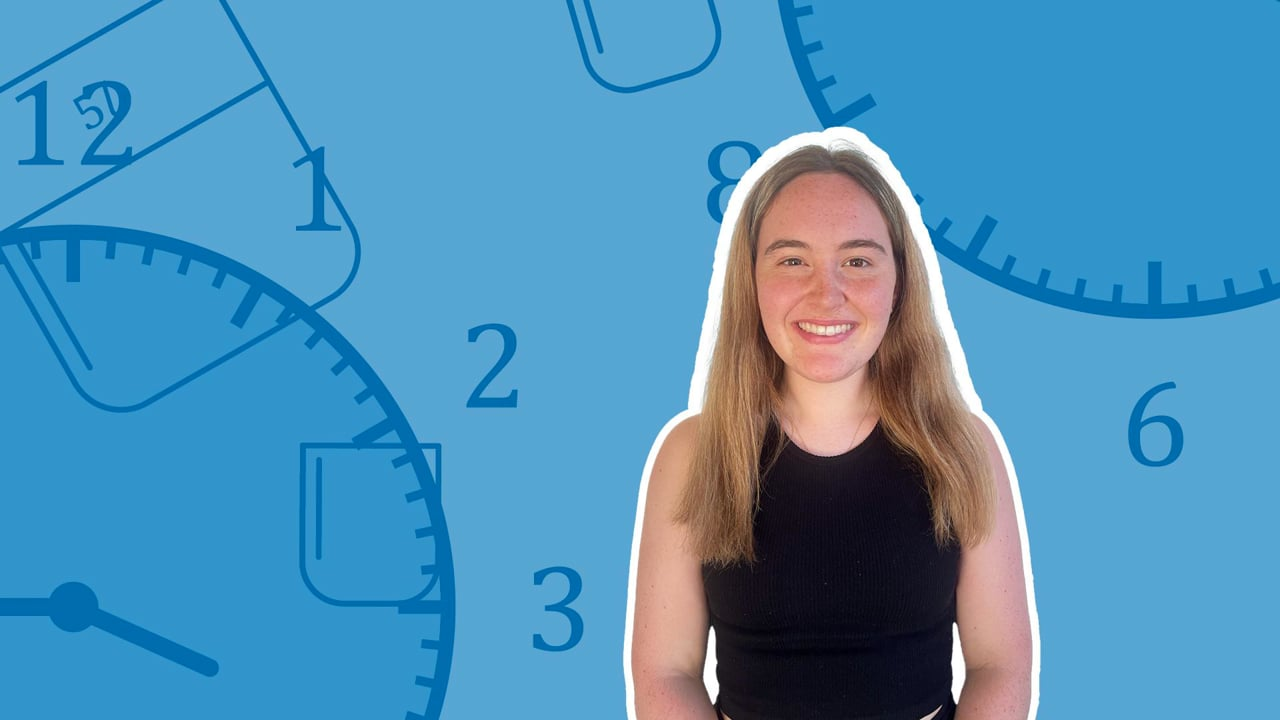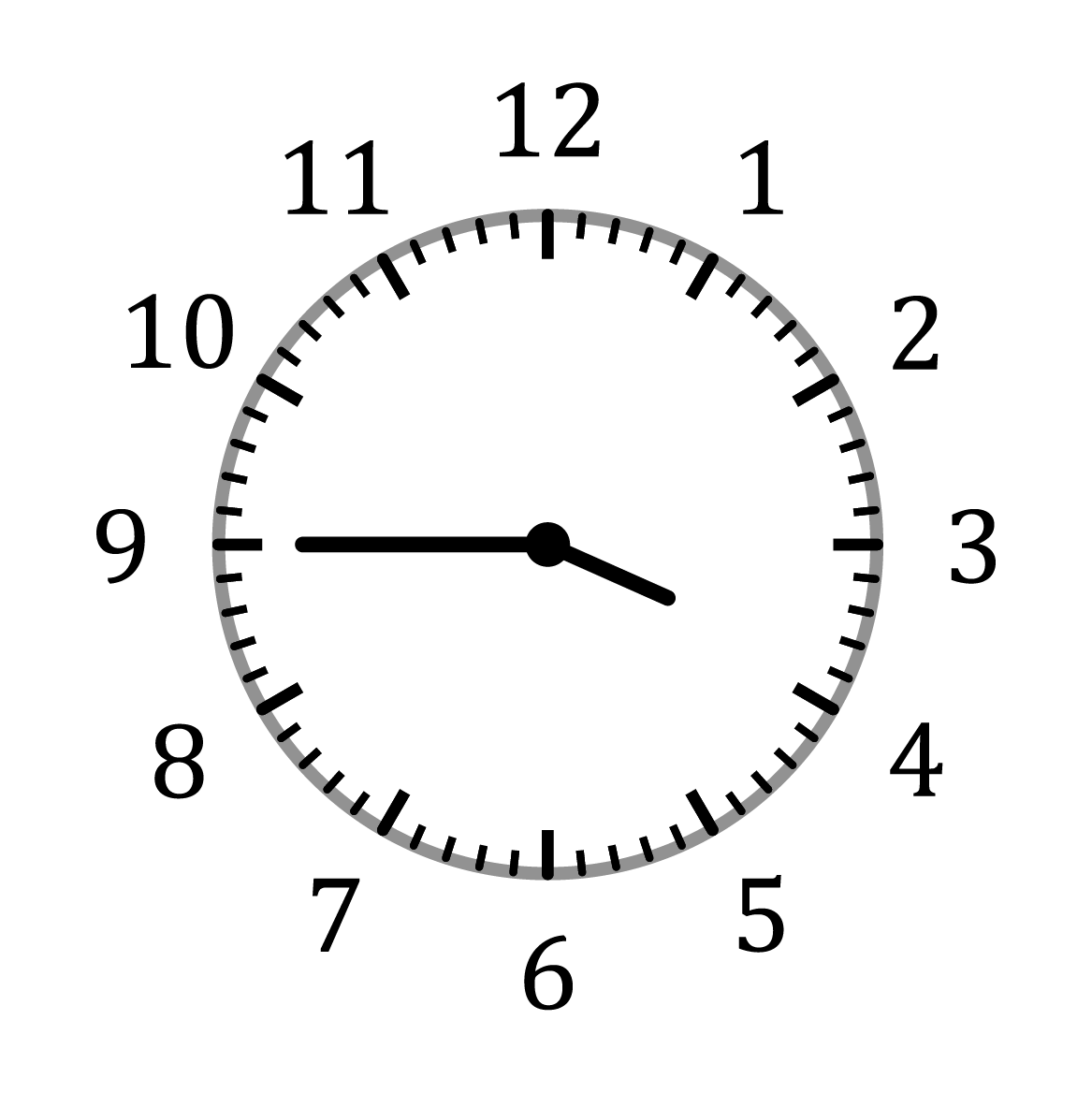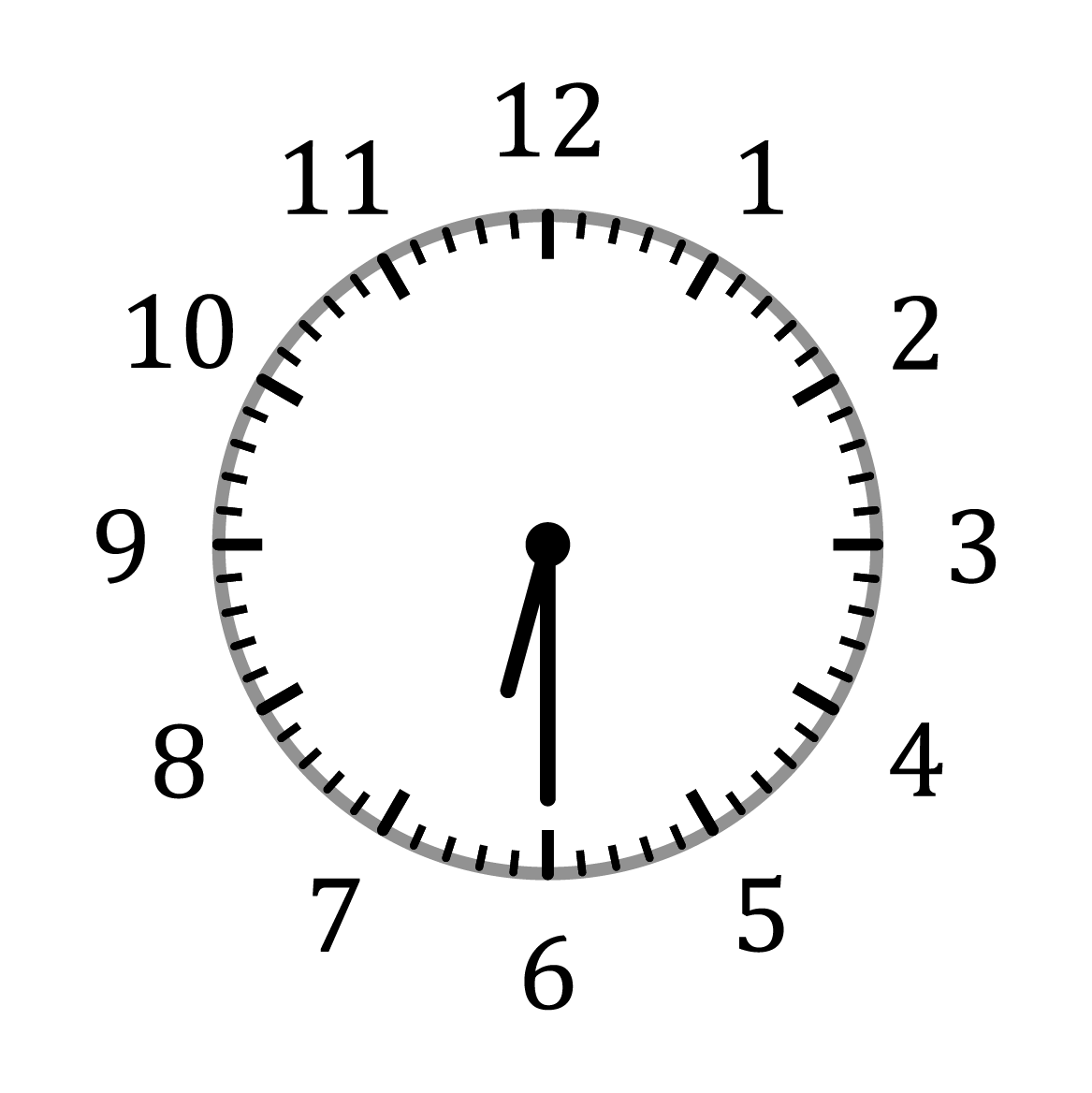Chapter overviewMaths

Number and place value

Multiplication and division

Fractions

Measurement

Geometry - properties of shapes

Statistics

Maths

# Telling the time0%

Summary

# Telling the time

## In a nutshell

The big hand of an analogue clock represents how many minutes have gone past the hour. The small hand represents which hour it is, and its angle shows how much of the hour has gone by. The thinnest hand shows how many seconds of the minute have gone by.

## Analogue clocks

An analogue clock has $3$ hands and a dial.

All hands move in the direction of the numbers from $1$ to $12$ - clockwise. The following table shows each hand, and what it does:

 Hand Size Description The hour hand Small The last hour which this hand has passed is the current hour. The minute hand Long, wide The last minute which this hand has passed is the current minute. The second hand Long, thin The line which this hand is pointing to is the current second of the minute.

On the dial, the features of the long lines and small lines with regards to the hands can be seen below:

 Line Hour hand Minute and second hand Long This shows which hour of the day it currently is Each long line represents 5 minutes of the hour, or 5 seconds of every minute. Short This has no relevance for the hour hand This shows the specific minute or second, up to 59 minutes or 59 seconds.

### Telling the time from an analogue clock

#### Procedure

 1 Read the hour; this is the last long line the hour hand has passed 2 Read the minute; this is the current line (long or short) which the minute hand is pointing at 3 (If required) Read the second; this is the current line (long or short) which the second hand is pointing at

Note: Some clocks use Roman numerals instead of numbers to represent the times $1 - 12$, or $\text{I } - \text{XII}$.  These clocks can be read the same way, but require you to understand how to read Roman numerals.

Certain phrases are also used to describe the time. These can be seen from the table below:

 Phrase Time Quarter past $15$​ minutes past Half past $30$​ minutes past Quarter to $45$​ minutes past / $15$​ minutes to

Typically for minutes between $0$ and $30$ the time is described as ... minutes past, and for minutes between $30$ and $60$ the time is described as $(60 - ...)$ minutes to.

##### Example 1

Write down the times shown from the following clocks:The first clock shows a time of $3$​ hours and $45$​ minutes, and so is $\underline{\text{a quarter to } 4}$.

The second clock shows a time of $6$​ hours and $30$​ minutes, and so is $\underline{\text{half past } 6}$​​.

## Digital clocks

Digital clocks do not have a dial nor hands, and they only show numbers to represent the time. The time is represented as HH:MM:SS where each pair shows the current hour, minute or second.

##### Example 2

What is the time shown on the following digital clock: 08:45:33?

The clock shows a time of $\underline{8 \text{ hours and } 45 \text{ minutes and } 33 \text{ seconds}}$.

On a $24$-hour clock, the hour varies between $0$ and $24$ with hours between $0$ and $12$representing the first half of the day, and hours between $12$ and $24$​ representing the later half of the day.

##### Example 3

What is the time shown on the following digital clock: 18:25:59?

$18 -12 =6$

Hence, the clock shows a time of $\underline{6 \text{ hours and } 25 \text{ minutes and } 59 \text{ seconds in the afternoon}}$.

​​

FAQs

• Question: What is an example of time?

Answer: Time is defined as the duration in which all things happen, or a precise instant that something happens. An example of time is breakfast at eight o'clock in the morning.

• Question: What are the two main ways of showing the time?

Answer: There are two different ways in which you can tell time. Try to understand the manner in which time is expressed based on the two types of clocks, digital and analogue.

• Question: Why is it important to be able to tell time?

Answer: Being able to tell the time can help you determine whether you're running late or whether you have plenty of time to spare. It can help you catch a train, bus, or plane on time, and allows you to know if you're going to make it to an important get-together early or late.

Theory

Exercises